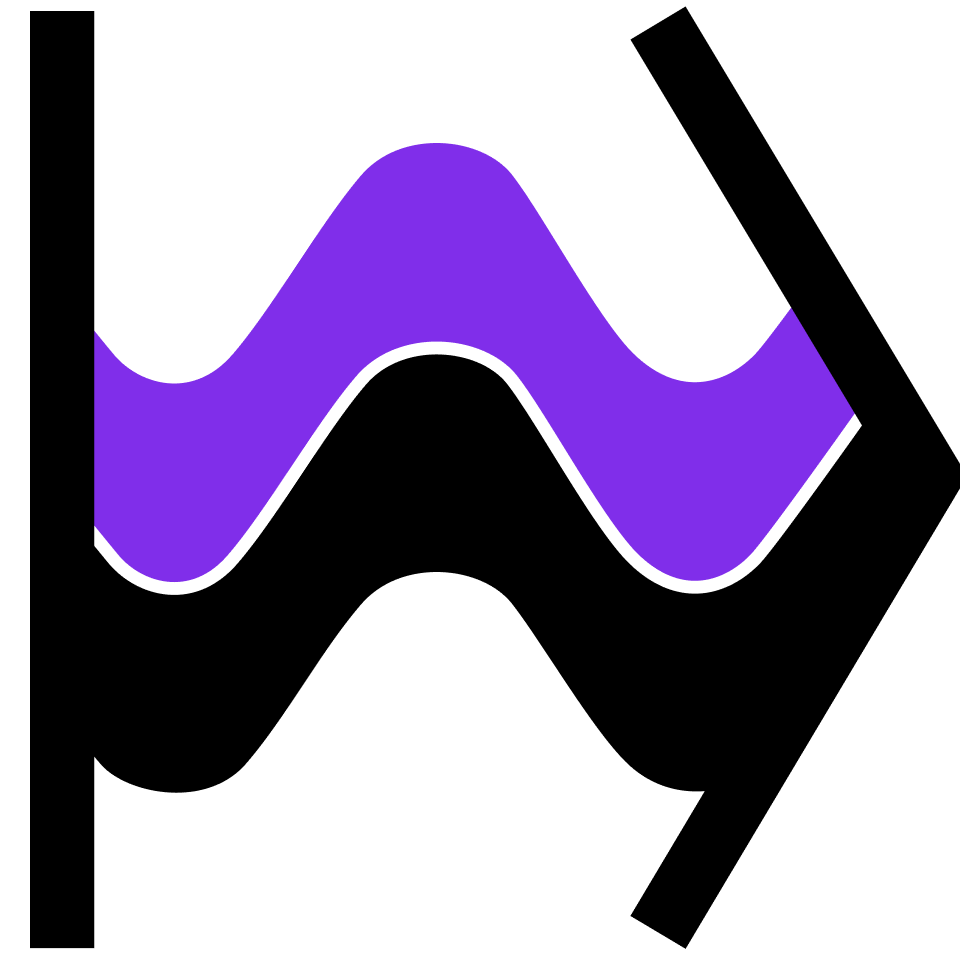## Linear Algebra with Applications

Delve into the abstract depths of linear algebra: vector spaces, determinants, eigenvalues, wedge products, and more.

What is a Vector?

Waves as Abstract Vectors

Why Vector Spaces?

The Gauss-Jordan Process I

The Gauss-Jordan Process II

Application: Markov Chains I

Real Euclidean Space I

Real Euclidean Space II

Span & Subspaces

Coordinates & Bases

Matrix Subspaces

Application: Coding Theory

Application: Graph Theory I

What Is a Matrix?

Linear Transformations

Matrix Products

Matrix Inverses

Application: Image Compression I

Application: Cryptography

Bivectors

Trivectors & Determinants

Determinant Properties

Multivector Geometry

Dual Space

Tensors & Forms

Tensor Products

Wedges & Determinants

Application: Markov Chains II

Eigenvalues & Eigenvectors

Diagonalizability

Normal Matrices

Jordan Normal Form

Application: Graph Theory II

Application: Discrete Cat Map

Application: Arnold's Cat Map

Inner Product Spaces

Gram-Schmidt Process

Least Squares Regression

Singular Values & Vectors

Singular Value Decompositions

SVD Applications

### Course description

Linear algebra plays a crucial role in many branches of applied science and pure mathematics. This course covers the core ideas of linear algebra and provides a solid foundation for future learning. Using geometric intuition as a starting point, the course journeys into the abstract aspects of linear algebra that make it so widely applicable. By the end you'll know about vector spaces, linear transformations, determinants, eigenvalues & eigenvectors, tensor & wedge products, and much more. The course also includes applications quizzes with topics drawn from such diverse areas as image compression, cryptography, error coding, chaos theory, and probability.

### Topics covered

• Bases
• Determinants
• Diagonalizable Matrices
• Eigenvalues and Eigenvectors
• Gaussian Elimination
• Gram-Schmidt Process
• Inner Products
• Inverses
• Linear Independence
• Linear Transformations
• Matrices
• Subspaces
• Tensors & Tensor Products
• Vector Spaces

### Prerequisites and next steps

A basic understanding of calculus and linear equations is necessary.

### Prerequisites

• Introduction to Linear Algebra
• Calculus in a Nutshell